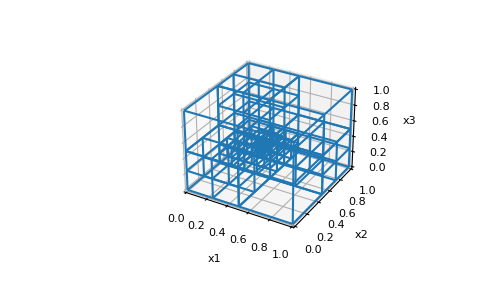# discretize.CylindricalMesh.plot_grid¶

CylindricalMesh.plot_grid(ax=None, nodes=False, faces=False, centers=False, edges=False, lines=True, show_it=False, **kwargs)[source]

Plot the grid for nodal, cell-centered and staggered grids.

For 2D and 3D meshes, this method plots the mesh grid. Additionally, the user can choose to denote edge, face, node and cell center locations. This function is built upon the `matplotlib.pyplot.plot` function and will accept associated keyword arguments.

Parameters
ax`matplotlib.axes.Axes` or `None`, `optional`

The axes to draw on. None produces a new axes.

nodes, faces, centers, edges, linesbool, `optional`

Whether to plot the corresponding item

show_itbool, `optional`

whether to call plt.show()

color`Color` or `str`, `optional`

If lines=True, defines the color of the grid lines.

linewidth`float`, `optional`

If lines=True, defines the thickness of the grid lines.

Returns
`matplotlib.axes.Axes`

Axes handle for the plot

Other Parameters
edges_x, edges_y, edges_z, faces_x, faces_y, faces_zbool, `optional`

When plotting a `TreeMesh`, these are also options to plot the individual component items.

cell_linebool, `optional`

When plotting a `TreeMesh`, you can also plot a line through the cell centers in order.

slice{‘both’, ‘theta’, ‘z’}

When plotting a `CylindricalMesh`, which dimension to slice over.

Notes

Excess arguments are passed on to plot

Examples

Plotting a 2D TensorMesh grid

```>>> from matplotlib import pyplot as plt
>>> import discretize
>>> import numpy as np
>>> h1 = np.linspace(.1, .5, 3)
>>> h2 = np.linspace(.1, .5, 5)
>>> mesh = discretize.TensorMesh([h1, h2])
>>> mesh.plot_grid(nodes=True, faces=True, centers=True, lines=True)
>>> plt.show()
```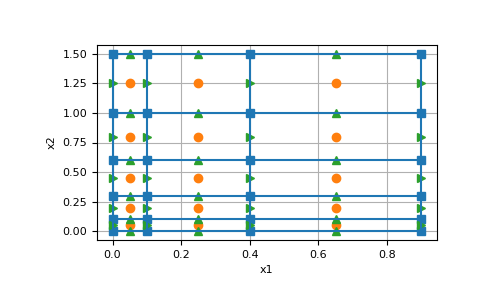Plotting a 3D TensorMesh grid

```>>> from matplotlib import pyplot as plt
>>> import discretize
>>> import numpy as np
>>> h1 = np.linspace(.1, .5, 3)
>>> h2 = np.linspace(.1, .5, 5)
>>> h3 = np.linspace(.1, .5, 3)
>>> mesh = discretize.TensorMesh([h1, h2, h3])
>>> mesh.plot_grid(nodes=True, faces=True, centers=True, lines=True)
>>> plt.show()
```

(png, pdf)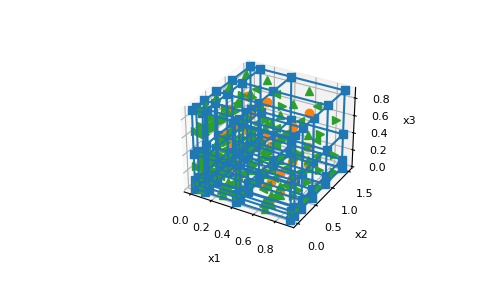Plotting a 2D CurvilinearMesh

```>>> from matplotlib import pyplot as plt
>>> import discretize
>>> X, Y = discretize.utils.exampleLrmGrid([10, 10], 'rotate')
>>> M = discretize.CurvilinearMesh([X, Y])
>>> M.plot_grid()
>>> plt.show()
```

(png, pdf)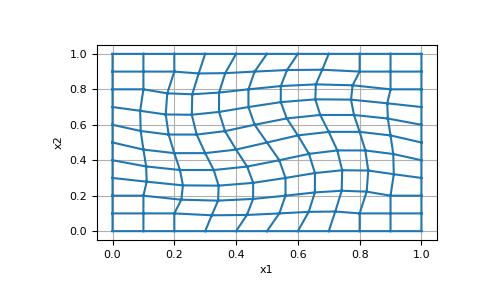Plotting a 3D CurvilinearMesh

```>>> from matplotlib import pyplot as plt
>>> import discretize
>>> X, Y, Z = discretize.utils.exampleLrmGrid([5, 5, 5], 'rotate')
>>> M = discretize.CurvilinearMesh([X, Y, Z])
>>> M.plot_grid()
>>> plt.show()
```

(png, pdf)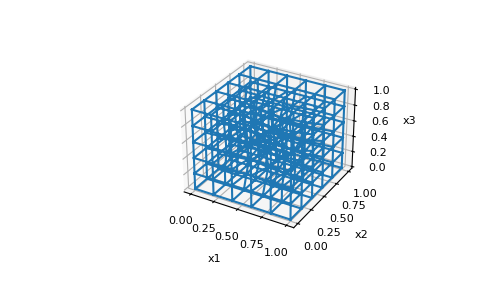Plotting a 2D TreeMesh

```>>> from matplotlib import pyplot as plt
>>> import discretize
>>> M = discretize.TreeMesh([32, 32])
>>> M.insert_cells([[0.25, 0.25]], )
>>> M.plot_grid()
>>> plt.show()
```

(png, pdf)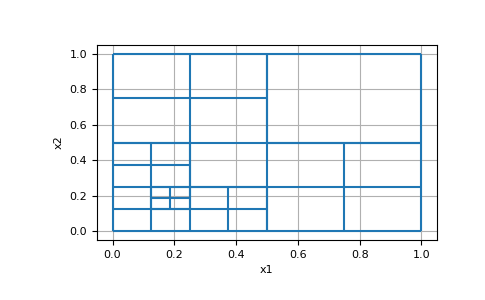Plotting a 3D TreeMesh

```>>> from matplotlib import pyplot as plt
>>> import discretize
>>> M = discretize.TreeMesh([32, 32, 32])
>>> M.insert_cells([[0.3, 0.75, 0.22]], )
>>> M.plot_grid()
>>> plt.show()
```

(png, pdf)